Скачать презентацию Simple Data Types Built-In and User Defined Chapter

f10a073ea8e77c6de316993cc1acc6ca.ppt

• Количество слайдов: 31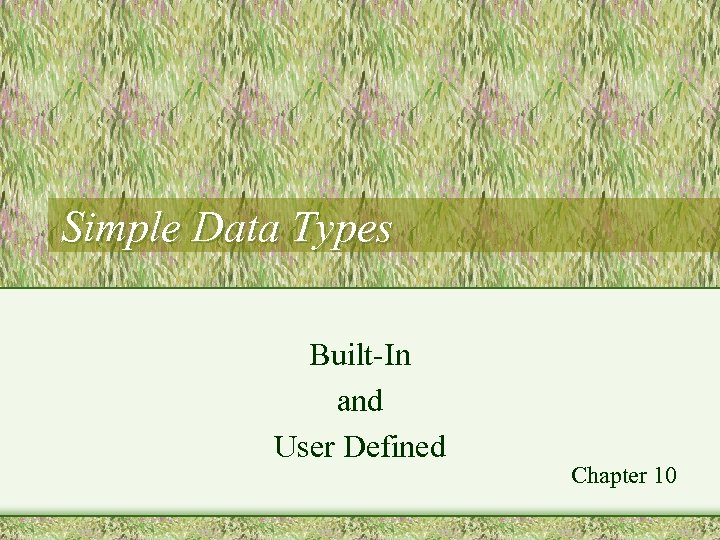Simple Data Types Built-In and User Defined Chapter 10Built-In Simple Types • Simple (or "atomic") • Each value is indivisible, a single entity • Examples – int, char, float • NOT simple types – strings, arrays, structs 2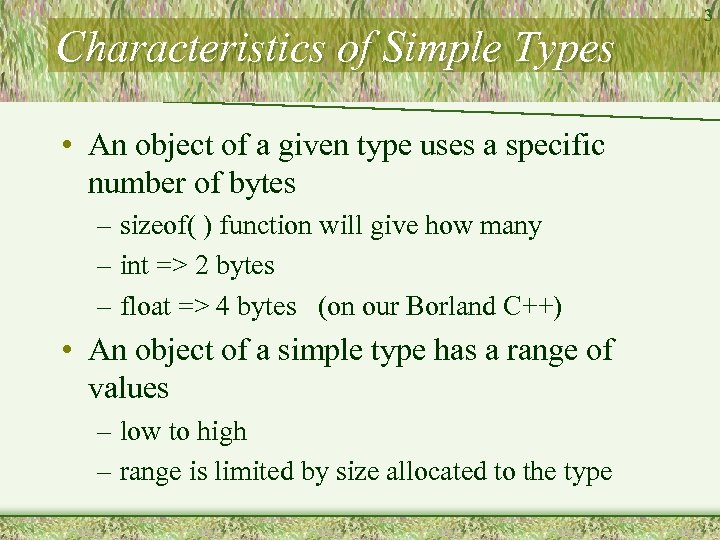Characteristics of Simple Types • An object of a given type uses a specific number of bytes – sizeof( ) function will give how many – int => 2 bytes – float => 4 bytes (on our Borland C++) • An object of a simple type has a range of values – low to high – range is limited by size allocated to the type 3Integral Types • Include – char, short, int, long – can be signed or unsigned – signed integer stored in 2 bytes – range is -32768 … 32767 • Constants can be specified in – decimal, octal, hex – normally in decimal 4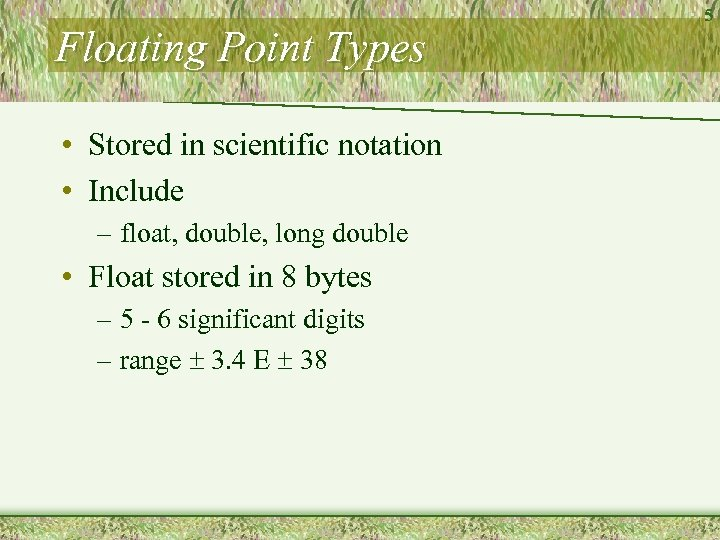Floating Point Types • Stored in scientific notation • Include – float, double, long double • Float stored in 8 bytes – 5 - 6 significant digits – range 3. 4 E 38 5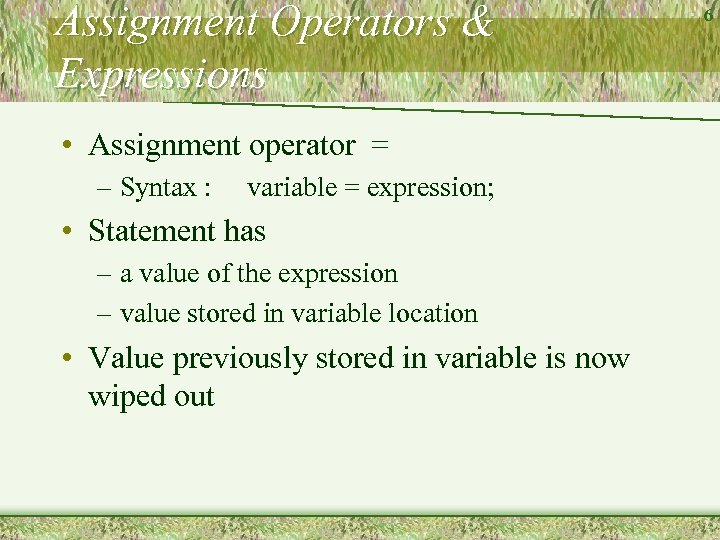Assignment Operators & Expressions • Assignment operator = – Syntax : variable = expression; • Statement has – a value of the expression – value stored in variable location • Value previously stored in variable is now wiped out 6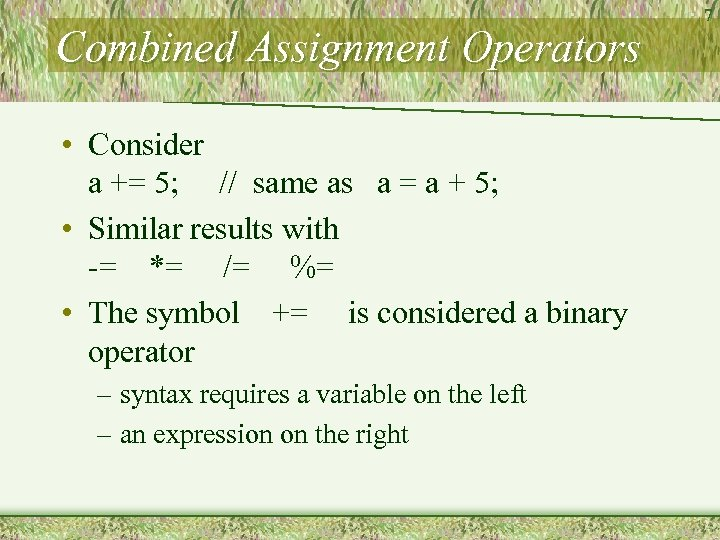Combined Assignment Operators • Consider a += 5; // same as a = a + 5; • Similar results with -= *= /= %= • The symbol += is considered a binary operator – syntax requires a variable on the left – an expression on the right 7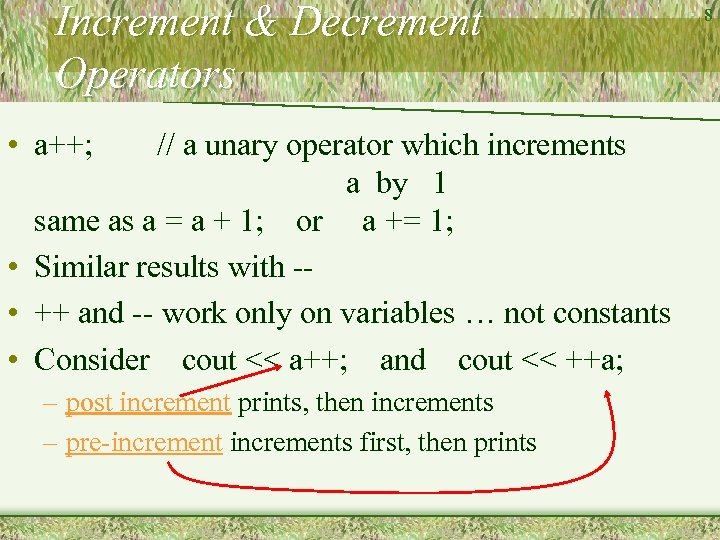Increment & Decrement Operators • a++; // a unary operator which increments a by 1 same as a = a + 1; or a += 1; • Similar results with - • ++ and -- work only on variables … not constants • Consider cout << a++; and cout << ++a; – post increment prints, then increments – pre-increments first, then prints 8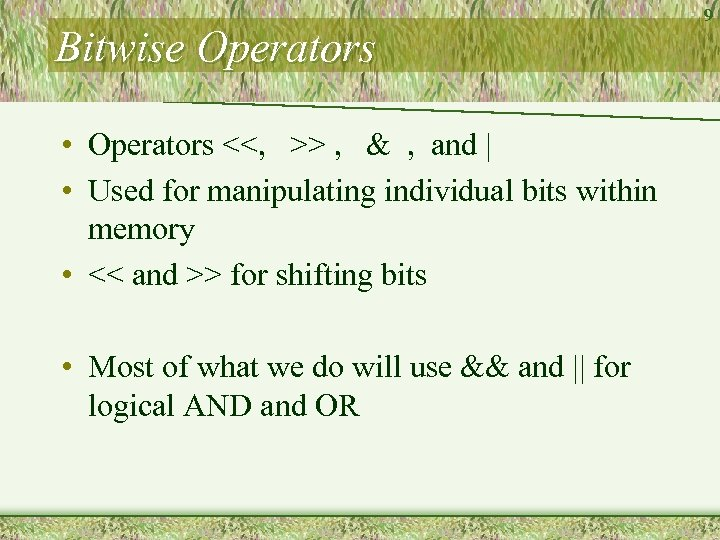Bitwise Operators • Operators <<, >> , & , and | • Used for manipulating individual bits within memory • << and >> for shifting bits • Most of what we do will use && and || for logical AND and OR 9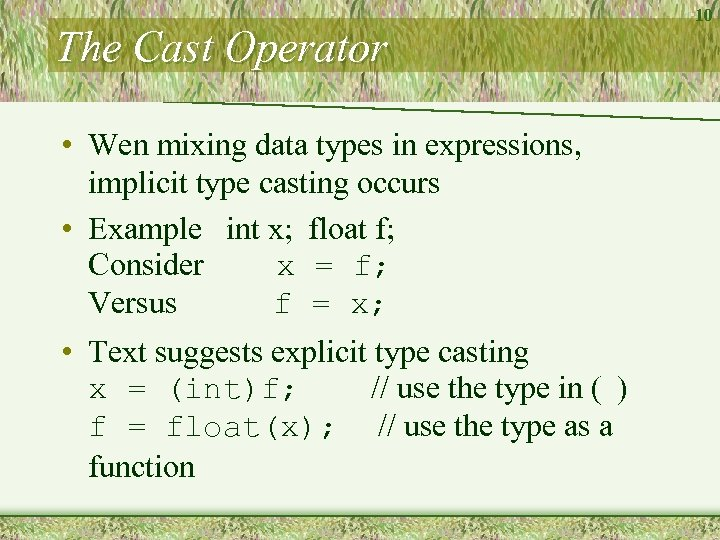The Cast Operator • Wen mixing data types in expressions, implicit type casting occurs • Example int x; float f; Consider x = f; Versus f = x; • Text suggests explicit type casting x = (int)f; // use the type in ( ) f = float(x); // use the type as a function 10The sizeof Operator • A unary operator – yields size (in bytes) of some variable or data type – almost acts as a function • cout << sizeof (part_struct); • Often used for arrays or structs where you need to specify number of bytes to be read in or sent to a file 11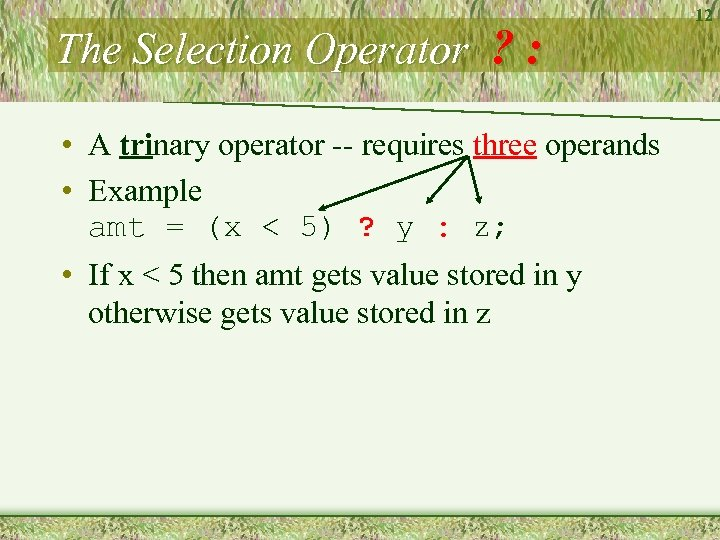The Selection Operator ? : • A trinary operator -- requires three operands • Example amt = (x < 5) ? y : z; • If x < 5 then amt gets value stored in y otherwise gets value stored in z 12Operator Precedence • Similar to algebraic precedence – Parentheses, unary, mult, div, addn, subt – Note page A 1 Appendix B • Note also that some are L-> R, others are R-> L: 13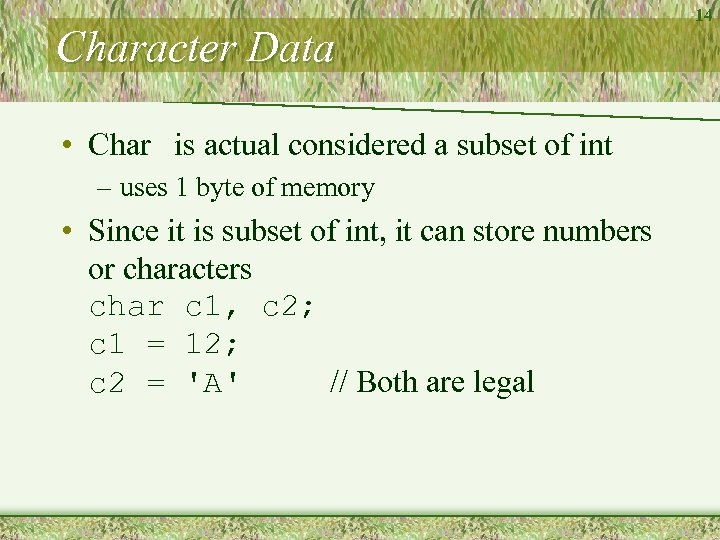Character Data • Char is actual considered a subset of int – uses 1 byte of memory • Since it is subset of int, it can store numbers or characters char c 1, c 2; c 1 = 12; c 2 = 'A' // Both are legal 14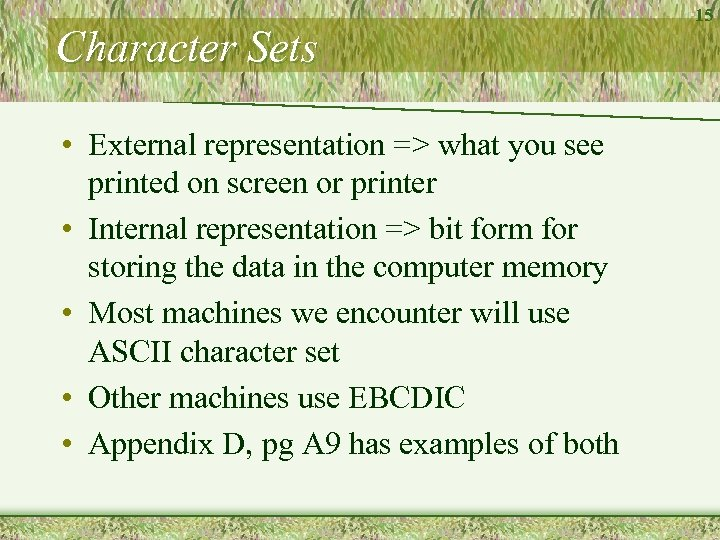Character Sets • External representation => what you see printed on screen or printer • Internal representation => bit form for storing the data in the computer memory • Most machines we encounter will use ASCII character set • Other machines use EBCDIC • Appendix D, pg A 9 has examples of both 15C++ char Constants • Single printable character enclosed by single quotes 'A' '7' '\$' – can be letters, numerals, or symbols • Control characters (non printable characters) used to control output 'n' for newline (same as endl) 'f' form feed 't' for tab 'a' for beep 16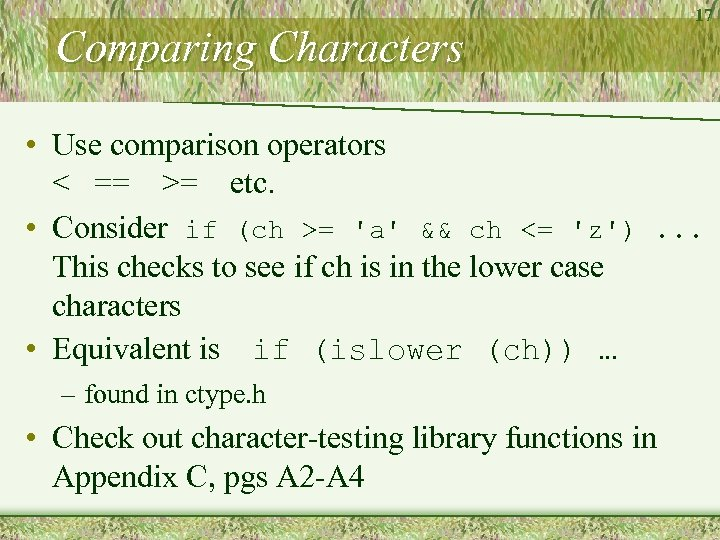Comparing Characters 17 • Use comparison operators < == >= etc. • Consider if (ch >= 'a' && ch <= 'z'). . . This checks to see if ch is in the lower case characters • Equivalent is if (islower (ch)) … – found in ctype. h • Check out character-testing library functions in Appendix C, pgs A 2 -A 4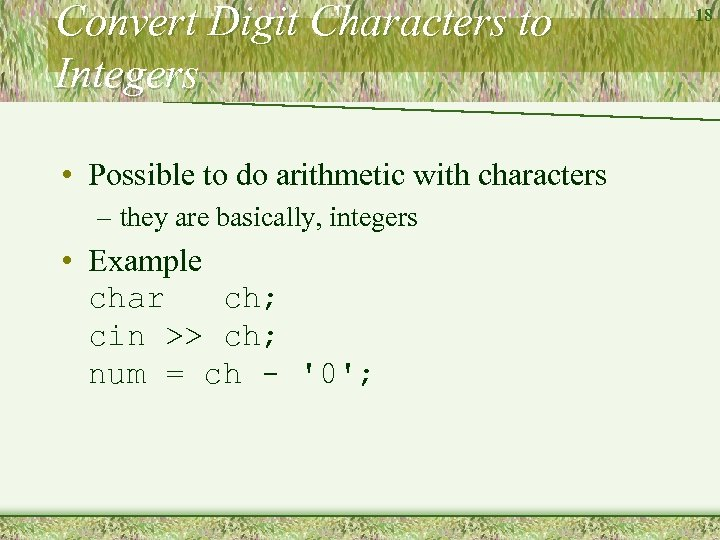Convert Digit Characters to Integers • Possible to do arithmetic with characters – they are basically, integers • Example char ch; cin >> ch; num = ch - '0'; 18Converting Lowercase to Uppercase • From ctype. h header file, use functions provided ch = toupper (ch); ch = tolower(ch): • Change only made if ch "needs" to be changed – if it is already uppercase, toupper( ) does nothing 19Representing Floating Point Numbers • Precision of 5 or 6 significant digits • Represented internally in scientific notation Four bytes will store – sign bit for the number – 5 or 6 significant digits – sign bit for the power – 38 range for the power 20Arithmetic with Floating Point Numbers • May lose accuracy due to round off error – especially when combining very large with very small numbers • Warnings – don't use floats to control loops – don't compare floats for equality, rather compare for closeness if ( abs (a - b) < 0. 0001) … 21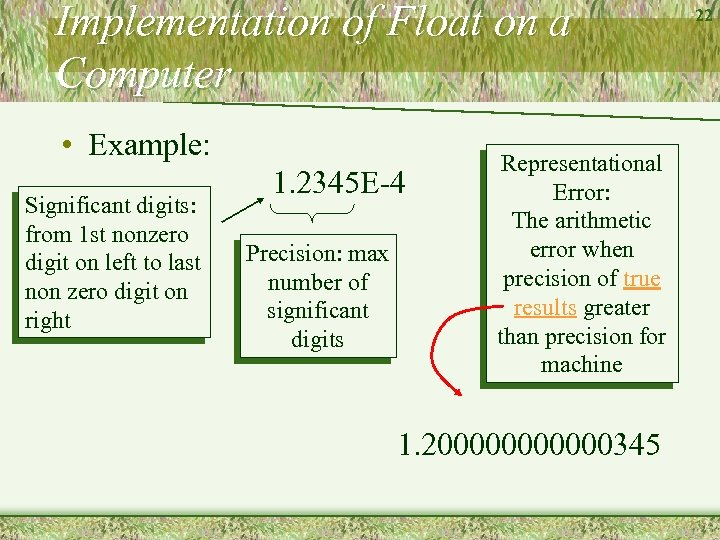Implementation of Float on a Computer • Example: Significant digits: from 1 st nonzero digit on left to last non zero digit on right 1. 2345 E-4 Precision: max number of significant digits Representational Error: The arithmetic error when precision of true results greater than precision for machine 1. 2000000345 22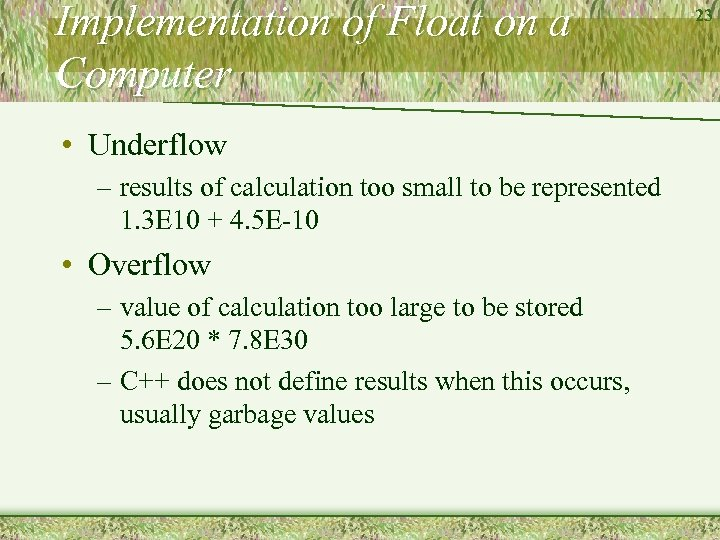Implementation of Float on a Computer • Underflow – results of calculation too small to be represented 1. 3 E 10 + 4. 5 E-10 • Overflow – value of calculation too large to be stored 5. 6 E 20 * 7. 8 E 30 – C++ does not define results when this occurs, usually garbage values 23The Typedef Statement • Syntax: typedef existing_type_name new_type_name; • Example: typedef int Boolean; • Does not really create a new type – is a valuable tool for writing self-documenting programs 24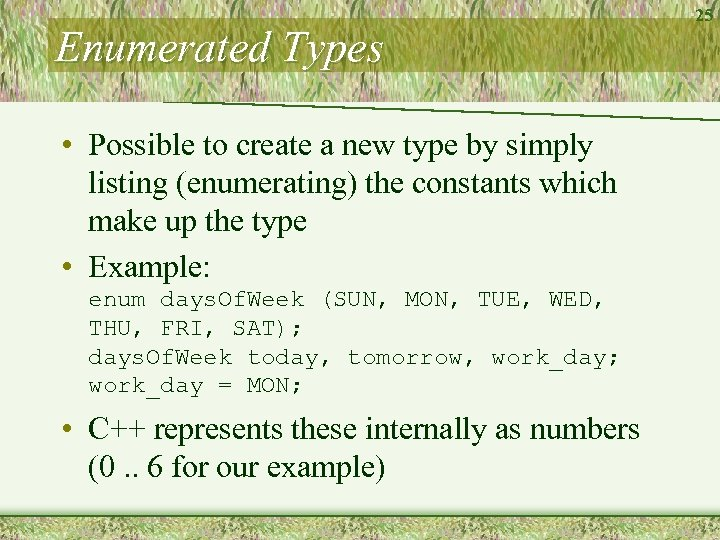Enumerated Types • Possible to create a new type by simply listing (enumerating) the constants which make up the type • Example: enum days. Of. Week (SUN, MON, TUE, WED, THU, FRI, SAT); days. Of. Week today, tomorrow, work_day; work_day = MON; • C++ represents these internally as numbers (0. . 6 for our example) 25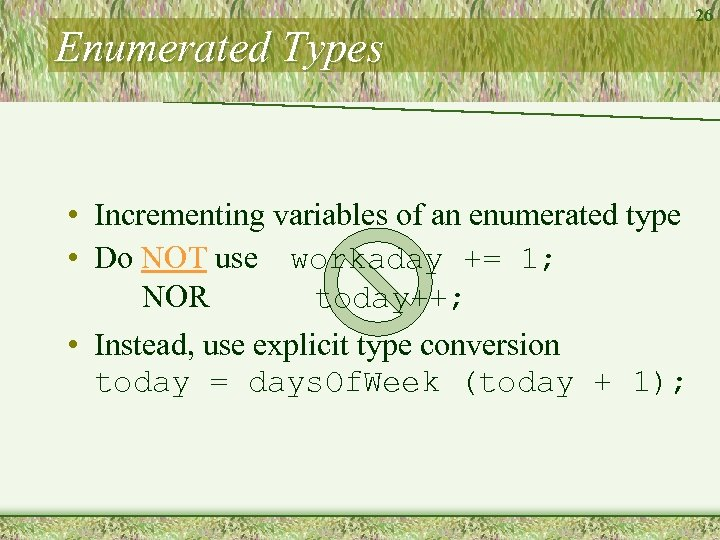Enumerated Types • Incrementing variables of an enumerated type • Do NOT use workaday += 1; NOR today++; • Instead, use explicit type conversion today = days. Of. Week (today + 1); 26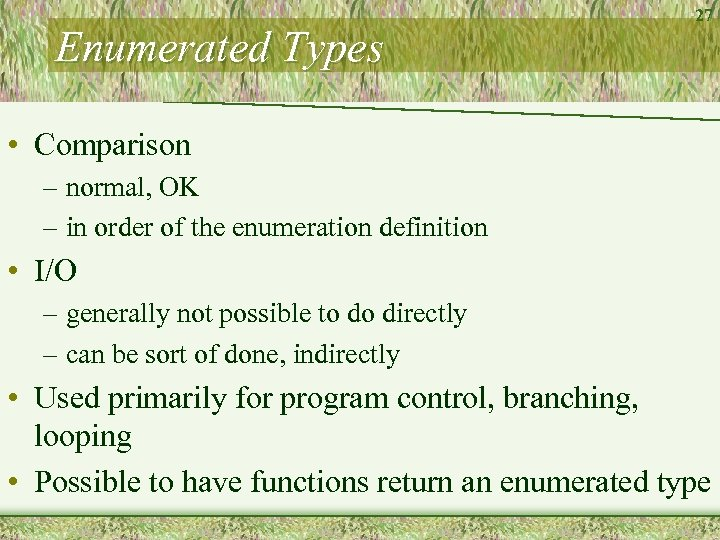Enumerated Types 27 • Comparison – normal, OK – in order of the enumeration definition • I/O – generally not possible to do directly – can be sort of done, indirectly • Used primarily for program control, branching, looping • Possible to have functions return an enumerated type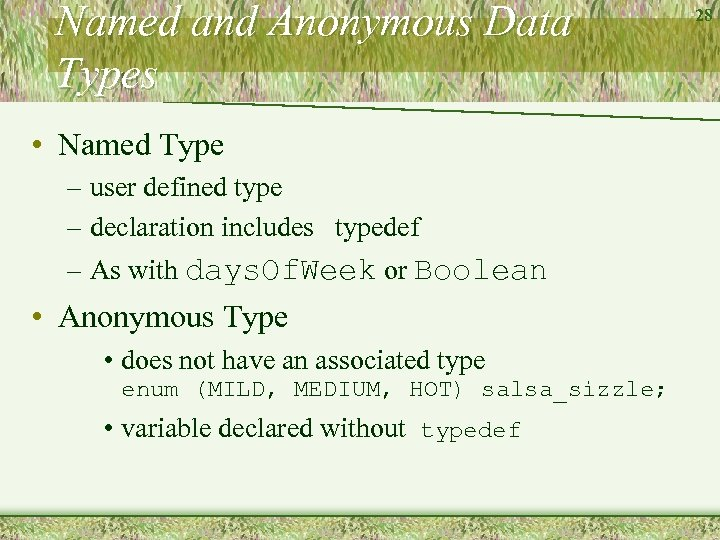Named and Anonymous Data Types • Named Type – user defined type – declaration includes typedef – As with days. Of. Week or Boolean • Anonymous Type • does not have an associated type enum (MILD, MEDIUM, HOT) salsa_sizzle; • variable declared without typedef 28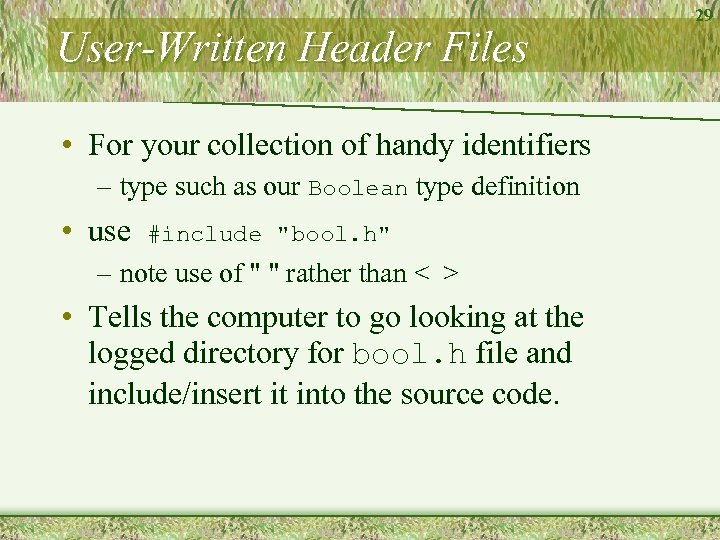User-Written Header Files • For your collection of handy identifiers – type such as our Boolean type definition • use #include "bool. h" – note use of " " rather than < > • Tells the computer to go looking at the logged directory for bool. h file and include/insert it into the source code. 29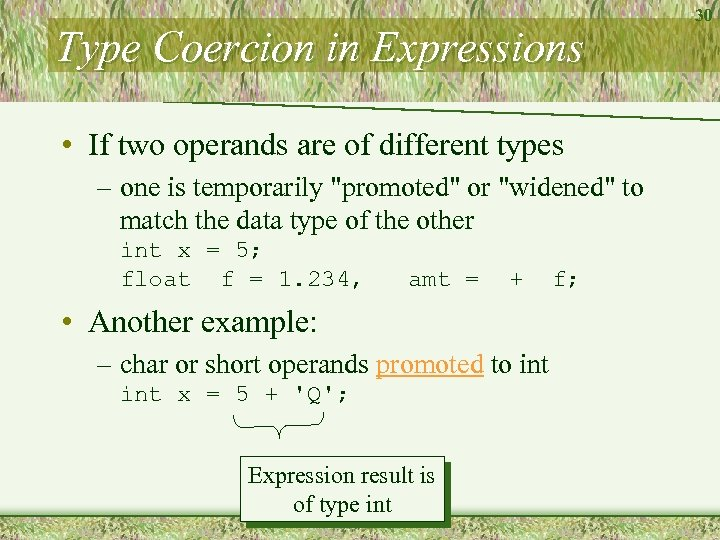Type Coercion in Expressions • If two operands are of different types – one is temporarily "promoted" or "widened" to match the data type of the other int x = 5; float f = 1. 234, amt = + • Another example: – char or short operands promoted to int x = 5 + 'Q'; Expression result is of type int f; 30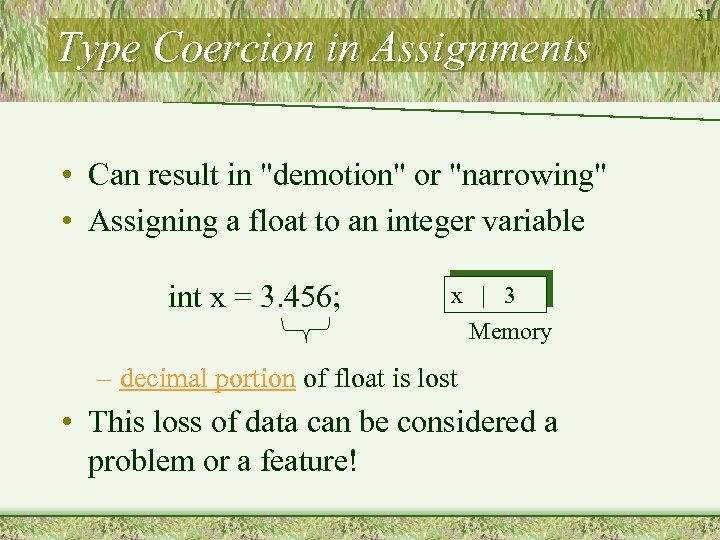Type Coercion in Assignments • Can result in "demotion" or "narrowing" • Assigning a float to an integer variable int x = 3. 456; x | 3 Memory – decimal portion of float is lost • This loss of data can be considered a problem or a feature! 31